9L Quick Quiz

9La

1       Why is it easier to cut something with a sharp knife than with a blunt one?

A       The area of the knife edge is large so pressure is greater.

B       The area of the knife edge is small so pressure is greater.

C       The area of the knife edge is large so pressure is smaller.

D       The area of the knife edge is small so pressure is smaller.

2       If all these birds are the same weight, which bird has the lowest pressure under its feet?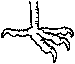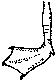A  crow B  seagull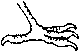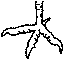C  raven D  chicken

3       Which is not a unit for pressure?

A       pascals

B       newtons per square metre

C       kilograms

D       newtons per square millimetre

4       A basketball player weighs 500 N. The area of one of his trainers is 250 cm2. When he puts all his weight on one foot, how much pressure does he put on the ground?

A       0.5 N/cm2                      B       2 N/cm2

C       250 N/cm2                     D      125 000 N/cm2

9Lb

1       The pressure in liquids and gases is caused by:

A       the particles pressing down.

B       the particles pushing up.

C       the particles moving sideways.

D       the particles moving in all directions and colliding with things.

2       Why does a dam at the end of a lake need to be wider at the bottom?

A       Water pressure is greater near the surface of the water.

B       There is more water pressure at the bottom of the lake.

C       To use up more concrete.

D       Water pressure decreases with depth.

3       When a gas is compressed:

A       the particles bump into the walls of the container less often.

B       the pressure drops.

C       the particles become more spread out.

D       the pressure increases.

4       A hydraulic system:

A       uses air to smooth out bumps.

B       uses air to send a force from one place to another.

C       uses a liquid to send a force from one place to another.

D       uses a solid to send a force from one place to another.

9Lc

1       In science, a lever is:

A       a complex machine which keeps the size of force you put in the same.

B       a simple machine which changes the size of force you put in.

C       a simple machine which changes the area of force you put in.

D       someone about to finish school.

2       Which are the correct labels for the lever shown below?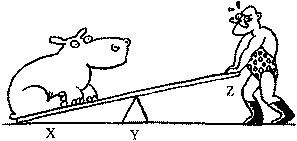A       X = effort, Y = pivot, Z = load

B       X = pivot, Y = effort, Z = load

C       X = load, Y = pivot, Z = effort

D       X = load, Y = effort, Z = pivot

3       How many diagrams show levers in action?

A       1            B       2            C       3            D       44       Which lever will make it easiest to move the rock?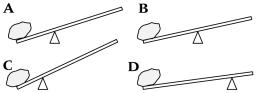9Ld

1       The moment of a force is:

A       its turning effect.

B       how long it takes to work.

C       how far it moves.

D       the distance of a force from a pivot.

2       You are opening a can of paint with a screwdriver. You apply a force of 10 N, 20 cm from the pivot. What is the moment of the force?

A       2 Nm                             B       20 Nm

C       200 Nm                         D      2000 Nm

3       What is a seesaw that is ‘in equilibrium’?

A       a balanced seesaw

B       a seesaw with the same weight on each side

C       a seesaw with both people the same distance from the middle

D       a wooden seesaw

4       What is the distance from the pivot of the boy on the seesaw?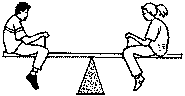Boy: 300 N ´ ? m           Girl: 450 N ´ 2 m

A       1 m                                B       2 m            C         3 m      D         4 m